## Selina ICSE Solutions for Class 9 Chemistry – Atomic Structure

Selina ICSE Solutions for Class 9 Chemistry Chapter 7 Atomic Structure

Exercise 7

Solution 1.

The latest research on atom has proved that most of the postulates of Dalton’s atomic theory contradict. But Dalton was right that atoms take part in chemical reactions. Comparison of Dalton’s atomic theory with Modem atomic theory.

 Dalton’s atomic theory Modern atomic theory 1. Atoms are indivisible particles. 1. Atoms are divisible into sub-atomic particles like protons, neutrons and electrons. 2. Atoms can neither be created nor destroyed. 2. Atoms can be created and destroyed by nuclear fusion and fission. 3. The atoms of an element are alike in all respect and differ from atoms of other elements. 3. The atoms of an element may not be alike in all respects, as it is seen in the case of isotopes. Isotopes which are atoms of the same element having the same atomic number but different mass numbers.

Solution 2.

(a) Inert elements: The elements which have complete outer most shell i.e. 2 or electrons. They ordinarily do not enter into any reaction.

(b) These exist as monoatoms because molecules of these elements contain only one atom.

(c) Valence electrons: The number of electrons present in the outermost shell or valence shell is known as valence electrons.

Solution 3.

The three isotopes of hydrogen differ only due to their mass number which is respectively 1,2 and 3 and named protium, deuterium and tritium.Solution 4.

 Atomic number Name with valency 4 – 15 A solid non-metal of valency 3. 8 A gas of valency 2. 19 A metal of valency 1. 14 A non-metal of valency 4.

Solution 5.

 Atom Atomic number Atomic mass No. of Protons No. of Electrons No. of Neutrons Electronic configuration (a) Sodium 11 23 11 11 12 2, 8, 1 (b) Chlorine 17 35 17 17 18 2, 8, 7 (c) Oxygen 8 16 8 8 8 2, 6 (d) Carbon 6 12 6 6 6 2, 4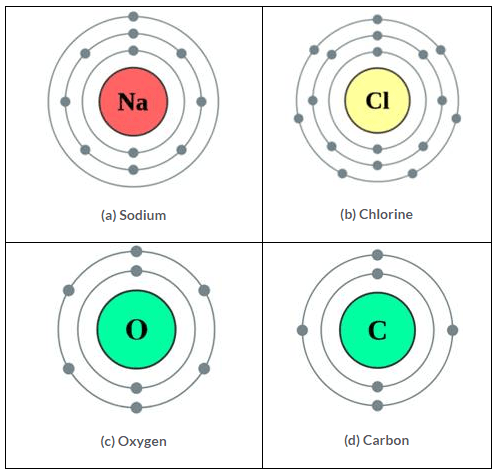Solution 6.

The significance of the number of protons found in the atoms in each of different element is fixed its place in periodic table.

Solution 7.

Atomic numbers of –

 X Y Z 6 9 12 (2,4) (2,7) (2,8,2)

(a) Y (2,7) forms Anion.
(b) Z (2, 8, 2) forms Cation.
(c) X (2,4) has four electrons in the valence shell.

Solution 8.

(a) X+1
(b) Oxidising agent, because it has ability to donate electron.

Solution 9.

(a) Mass number:The atomic mass number is defined as the sum of the number of protons and neutrons contained in the nucleus of an atom of that element. It is denoted by the symbol A.

(b) Ion: An atom or molecule that carries a positive or negative charge because of loss or gain of electrons.

(c) Cation: It is positively charged ion that is formed when an atom loses one or more electrons e.g. Na+, Hg2+, Ca2+ etc.

(d) Atom: It is defined as the smallest unit of matter which takes part in a chemical reaction.

(e) Element: It is a substance which cannot be split up into two or simpler substances by usual chemical methods of applying heat, light or electric energy. e.g. Hydrogen, Oxygen, Chlorine etc.

(f) Orbit: It is defined as a circular path around the nucleus in which electrons of the atom revolve.

Solution 10.

Atomic number = 2 Mass number = 4

Solution 11.

(a) (i) Atom E contains 7 protons.
(ii) Atom E has an electronic configuration 2, 7.

(b) Atom C Stands for 73Li
Atom D stands for 816O
Compound formula = Li2O

(c) Metals are: A and C Non-metals are: E, D, E

Solution 12.

No of electrons in M Shell = 2
Number of electrons in K and L shell will be 2, 8 and respectively.
Therefore, Electronic configuration will be: 2, 8, 2.
Atomic number = 2 + 8 + 2 = 12, Since, atomic number = Number of Protons
No of Protons = 12

Solution 13.(a) (ii) Electronic configuration = 2, 8, 2
(b) Mass numbers are different of two isotopes of magnesium because of different number of neutron, that is, 12 and 14 respectively.

Solution 14.

Nucleons: Particles which constitute nucleus are called nucleons. Proton and neutrons are the nucleons.
At. weight of phosphorus = 31 Atomic number = 15
Total number of nucleons = 31 (No. of P + No. of N) No. of neutrons = 31 – 15 = 16
Electronic configuration
No. of electrons = 15 = 2,8,5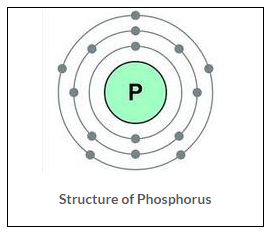Solution 15.

(a) Atoms of the same elements differing in the number of neutrons in their nuclei are known as isotopes. Thus, isotopes of an element have the same atomic number but different atomic mass number.
The fundamental particles is Neutrons which differs.

Uses of isotopes:

1. Some isotopes are Redioactive; isotopes of cobalt are used for treating cancer and other diseases.
2. An isotopes of uranium 235U is used as a fuel in nuclear reactor.

(b)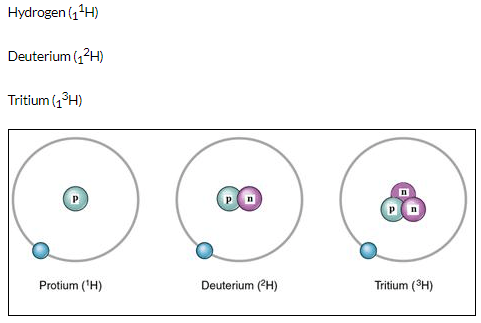Solution 16.

In chemical reactions only electrons take part. The chemical properties depend upon the electronic configuration.

The isotopes of element 1735Cl and 1737Cl have same atomic number and hence, the same configuration. So they have same chemical properties. These differ only in physical contents and weights because neutrons contribute to the mass of an atom.

1735Cl and 1737Cl have different number of neutrons 18 and 20 respectively.

Solution 17.

The atomic masses of the isotopes of chlorine are 35 and 37. However in any given sample of chlorine gas, the isotopes occur in approximate 3 : 1, 75% of Cl35, and 25% of Cl37. Therefore, the relative atomic mass or atomic weight of chlorine is 35.5.

Fractional atomic weight of chlorine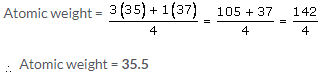Solution 18.

(a) Atomic number: The number of protons present in the nucleus of an atom is the atomic number of that atom. It is represented by the symbol Z.
Atomic number (Z) = Number of protons (p)

(b)

 No. of Protons No. of Electrons No. of Neutrons Atomic number Mass number 35 Cl 17 17 17 18 17 35 37 Cl 17 17 17 20 17 37

(c) Electronic configuration of chlorine is 2, 8, 7.

Solution 19.

(a) Hydrogen
(b) Element of zero group i.e. He (Helium)
(c) Calcium (2, 8, 8, 2). Therefore, 2 electrons in valance shell. Hence valency is 2.
(d) Chlorine atoms: 1735Cl and 1737Cl
(e) K shell.

Solution 20.

(a) Physical properties depend on the Atomic mass and isotopes have different mass number. (A) i.e. they have different number of neutrons. So, isotopes have different physical properties.

(b) Argon does not react as, Argon has completely filled outer-most orbit. The atomic number of argon is 18. Therefore, electronic configuration is 2, 8, 8. There are 8 electrons in the outermost or valance shell. Therefore, argon does not react.

(c) Actual Atomic Mass is greater than mass number (P + N) since mass number is a whole number approximation of atomic mass unit. In fact Neutrons are slightly heavier than protons and atom includes the existence of over 200 sub-atomic particles.

(d) 1735Cl and 1737Cl are isotopes of chlorine element which differ in number of neutrons. Whereas chemical properties are determined by electronic configuration of an atom. Isotopes of an element are chemically alike.

Solution 21.

Element A

Atomic number = 7
Electronic configuration: = 2, 5
Valency of element A = 8-5 = (3)

Element B

Electronic configuration 2,8,8 Valency of element B = Zero Element C
Number of Electrons 13 Electronic configuration: 2,8,3
Valancy of element C = 3+

Element D

Protons = 18 = electrons Electronic configuration: 2,8, 8
Valency of element D = Zero

Element E

Electronic configuration = 2, 8, 8, 1
Valency of element E = 1+
[ii] C and E are metals [iii] A is a Non-metal
[iv] B and D are inert gases. (A, C and E are not inert gases.)

Solution 22.

(a) C. Atomic nucleus
(b) A. 6
(c) C. 2,8,8,1

Solution 23.

Elements tend to combine with one another to attain the stable electronic configuration of the nearest noble gas/inert gas (Duplet or Octet)

(a) Sodium Chloride

The electronic configuration of Sodium is 2, 8, Thus, Sodium atom tends to lose one electron to attain the stable electronic configuration of neon gas 2, 8.

The electronic configuration of Chlorine is 2, 8, 7. Thus, Chlorine atom needs one electron to complete its octet and achieve the stable electronic configuration of Argon 2, 8, 8.

When sodium and chlorine atoms approach each other, the sodium atom, Na, loses an electron to form sodium ion, The cation, Na+, carries a single positive charge.
Na → Na+ + e

The electron lost by sodium atom is transferred to the chlorine atom forming chloride ion, Cl.
Cl + e → Cl

The sodium and chloride ions thus, achieve stable electronic configurations.(b) Hydrogen H2

Hydrogen atom has one electron in its valence shell. Hydrogen atom needs one more electron to complete its duplet and attain the stable electronic configuration of Helium.

In case of hydrogen H2 molecule, each of the two hydrogen H atoms contributes one electron so as to have one shared pair of electrons. Both the hydrogen atoms attain stable duplet structure resulting in the formation of a single covalent bond [H – H] between them.

Both the hydrogen atoms have equal attraction for the electrons. Thus, the shared pair of electrons remains equidistant from both the atoms.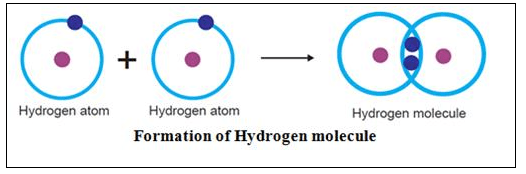Solution 24.

 Element Symbol Atomic Number Mass Number Number of Neutrons Number of Electrons Number of Protons Li 3 6 4 3 3 Cl 17 35 18 17 17 Na 11 23 12 12 11 Al 13 27 14 13 13 S 15 32 16 15 15